## General Mechanical

•peteroznewman
Subscriber

A linear statics model calculates a maximum equivalent stress, which is compared with a yield strength.

An initial mesh has a certain element size around the point of maximum stress.
A second mesh with smaller elements is solved and gives a new value of maximum stress.
A third mesh with even smaller elements gives a third value of maximum stress.

How do you decide when to stop refining?

One method is described in ASME V&V10.1 “Illustration on V&V for Computational Solid Mechanics” and is called Calculation Verification, one small part of the entire Verification and Validation process.

Below is an example using a tetrahedral mesh with a Sizing Mesh Control on a Sphere of Influence.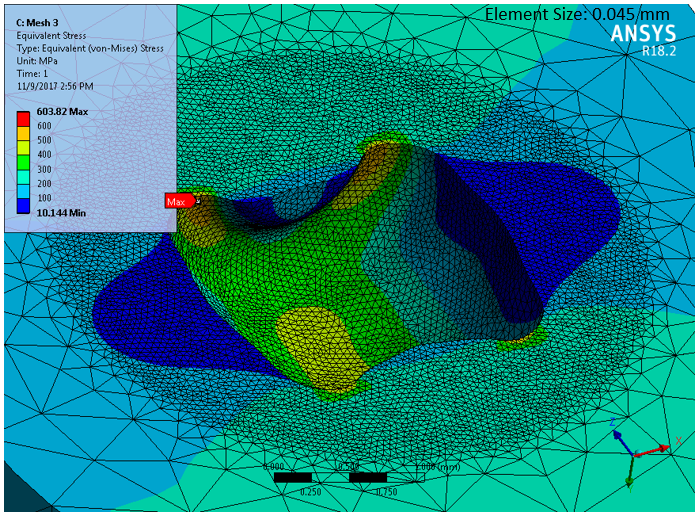Maximum Stress from six mesh sizes were plotted. The red line is a best fit line through the three smallest element sizes. This line, or the calculation in Section 7.2 of V&V10.1, can be used to extrapolate the maximum stress to a zero-size element. This is the estimate for the exact maximum stress, but is only valid when the results are being calculated in the asymptotic convergence regime. The line estimates the maximum stress is 607 MPa.  See this discussion for an alternative mesh control using inflation.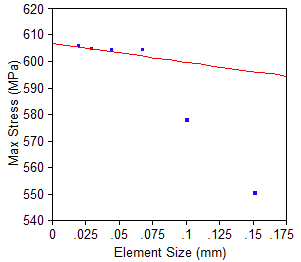The three largest element sizes are clearly trending toward a very different zero-size element value and should not be used since those results are not in the asymptotic convergence regime. V&V10.1 has more information on how to determine if you are in that regime.

In this example, I used a factor of 1.5 to change each successive element size. V&V10.1 recommends the factor be > 1.3.

•Rashi
Subscriber

In your article and in the V&V10.1 it mentions about "asymptotic convergence regime", how can we know our results are in this region?

The explanation in the standard is bit confusing (V&V10.1). Which compares a theoretical and a calculated value for variable"p".

•peteroznewman
Subscriber

Great question Rashi, and one that I had also pondered after finding the illustration and the standard to be lacking in clarity on that point.

Here is a tutorial that someone at NASA put together for CFD models. It has a paragraph on Asymptotic Range of Convergence.

•mekafime
Subscriber

Hi Peter,

for this example, is recommend use 0.1 or 0.15 element size?

•peteroznewman
Subscriber

Hi Mekafime,

The element size is not an absolute value, and it would depend on the length units anyway.

The idea is that you let ANSYS mesh with an edge size that makes a reasonable looking mesh to get your first point on the plot.

Say in your case, the element edge length was 12 mm.  The rule is to divide by 1.5 to get the next point on the plot. So the series of solutions for the mesh size for elements around the peak stress would be: 12, 8, 5.3, 3.5, 2.4, 1.6 mm

You solve for each of those element sizes and plot the corresponding Maximum Stress.  Once you start to get a set of three or four points that are trending toward a straight line, you can stop cutting the element size.

Regards,

Peter

•mekafime
Subscriber
•peteroznewman
Subscriber

Hi mekafime,

Yes, that may work if you are on an unlimited Research license, since you are making a global mesh size change, you will get smaller elements everywhere, even away from the peak stress where the smaller elements are not needed.

If you are on a Student license, you might find that you exceed the allowable node count of 32,000 nodes before you have enough points on your mesh convergence plot when using the above mesh refinement approach.  A more efficient method is to apply localized sizing around the peak stress. Create a Coordinate System near the location of peak stress. Create a Sizing control on the bodies and set the size using a Sphere of Interest with an appropriate radius so that the smaller elements are inside that sphere, while outside that sphere the global mesh size is used.

Sometimes a model includes a stress singularity, which means that the stress keeps increasing as the element size decreases. Adding a blend to a sharp interior corner of the geometry in CAD is the usual mitigation to eliminate the stress singularity.

Regards,

Peter

•DrAmine
Ansys Employee

Enough to deduce that your solution is mesh independent and you can then switch on to a Richardson extrapolation. Here some ideas from the NASA:

https://www.grc.nasa.gov/www/wind/valid/tutorial/spatconv.html

•mekafime
Subscriber

Hi Peter,

I just found a problem like the one you mentioned about the stress singularity as the size of the elements is reduced. How I can add a blend to a Sharp interior corner ? Have you a video about this?

Thanks!

•peteroznewman
Subscriber

How you add a blend depends on the Geometry editor you use. Is it DesignModeler, SpaceClaim or another CAD system?

•mekafime
Subscriber

Hi Peter

DesignModeler and SolidWorks.

•peteroznewman
Subscriber

In SolidWorks, you use the Fillet tool.

•mekafime
Subscriber
•peteroznewman
Subscriber

What is the wall thickness?   What displacement are you measuring?

I suggest the sphere of influence include the fillet around both tubes instead of just the center portion.

•mekafime
Subscriber
•peteroznewman
Subscriber

I suggest you forget about the Sphere of Influence and just use the Mesh sizing control that is applied to the two tubes and the fillet. You can try size 10, 6.6, 4.4, 3.0, 2.0, 1.3 mm and plot that data.  What would be interesting is to see the effect on the results at a fixed element size, say 3 mm, of changing the Formulation of the contact.  MPC is probably the best.

I'm concerned that you have bonded contact in the region of high plasticity.  I refer you back to the post that I made before that I don't like contact used in regions of high plasticity.  This image represents an alternative way to make the connection. The bonded contact is far from the region of high plasticity which is occurring in a contiguous solid.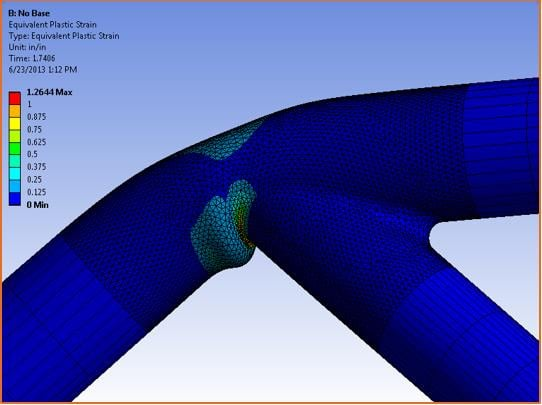•mekafime
Subscriber
•peteroznewman
Subscriber

Boolean Unite

•mekafime
Subscriber
•mekafime
Subscriber
•peteroznewman
Subscriber

Great, now you can do the element size series for all those bodies meshed at 10 mm, 6.6 mm, 4.4 mm, 3.0 mm, 2.0 mm, 1.3 mm element size and plot the result data.  If you are using plasticity, Equivalent Total Strain is a good result to plot.

•mekafime
Subscriber
•mekafime
Subscriber

Hi Peter

I already obtained the requested graphic but I have a problema in this (new) computer when I use 2 mm of element length the computer freezes and I have to restart (Do you know how I can solve that in ANSYS?). So I'm using 3 mm as minimum element size up to15 mm.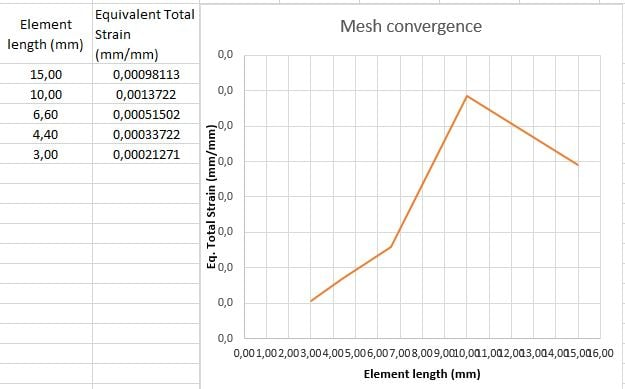•peteroznewman
Subscriber
•mekafime
Subscriber

•mekafime
Subscriber

Hi Peter,

Could you open the model or I attach it wrong?

•peteroznewman
Subscriber

I have opened your model in 2019 R2.

I see you measured Equivalent Total Strain at a Node.  The intention of the convergence check is to plot the maximum global value, not one specific node. I am running your model across the element sizes to get new data to plot. This is automated using Parameter Set.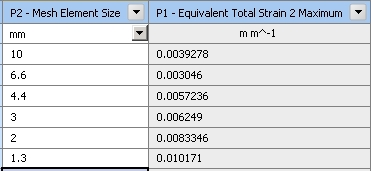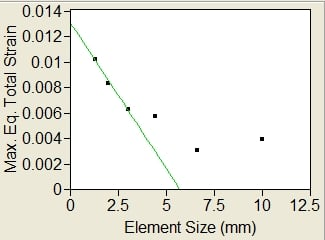Is this data evidence of convergence to a zero element size result of Max. Eq. Total Strain = 0.013 or would the next data point at 0.89 mm element size be much greater than that?  UPDATE: the result for element size 0.89 mm is 0.013265.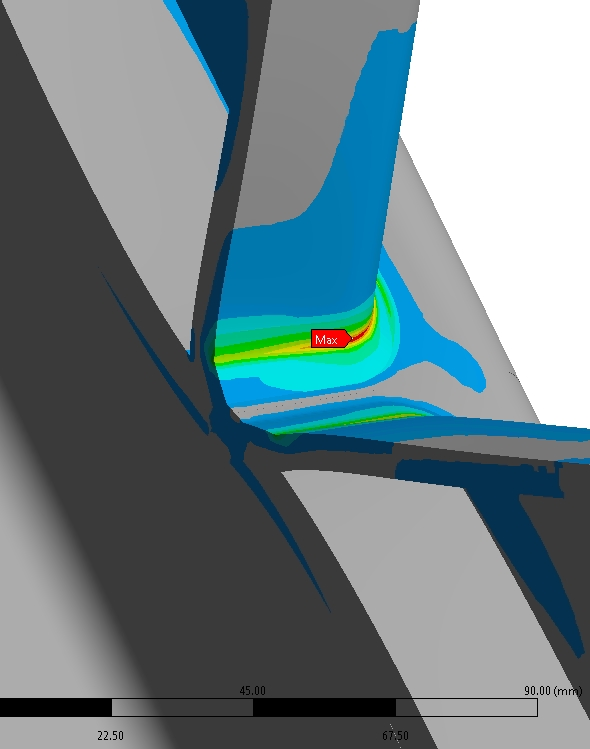•peteroznewman
Subscriber

I added the last point to the convergence plot.
The green line is the best fit through the last 4 points, the red line through the last 2 points.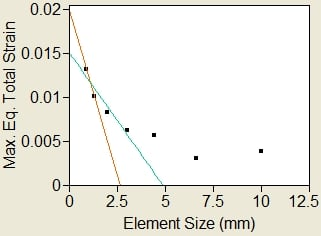It might be reasonable to estimate that the Max. Total Strain is < 2%.

•mekafime
Subscriber

Hi Peter,

I'm trying to reproduce the same values that you obtained, I have some doubst.

- How do I get the graph ? (I'm in parameter set)

- 1.3/1.5 = 0.866 (0.89) or do you refer to another value?

- Would the ideal size be 1 mm, how much more could I take?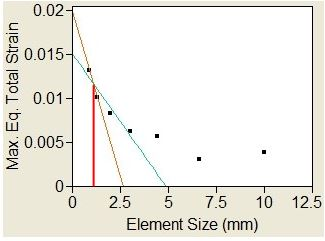•mekafime
Subscriber

I tried to parameterize the unión but the same thing happens to me when I use 2 mm of size of element the computer freezes, What other option do I have?

•peteroznewman
Subscriber

I was going from the 3, 2, 1.3333, 0.8888, 0.5925 progression and rounding off. I was tempted to run the last value to see where it came out. I switched to Sphere of Influence to keep the mesh size within the ability to solve In-core for the 0.89 mm size.

Suppose I did run the 0.59 mm size and it turned out to be on the green line pointing toward a Max. Eq. Total Stain of 0.02.  That means that if you ran the model with the 1.3 mm element size, the result would show a Max. Eq. Total Strain of 0.01 while the true solution was 0.02.  You could say that is a 100% error, but the better way to think of it is to compare the value with a critical value such as the elongation at break.

If your model was for a brittle material that has an elongation at break of 0.015, then the difference between a result of 0.01 and 0.02 is the difference between predicting success and predicting failure to support the load. In that case it would be important to use small elements.

If your model was for a ductile material that has an elongation at break of 0.5, then the difference between 0.01 and 0.02 is insignificant. In that case you could use larger elements.

•mekafime
Subscriber

Hi Peter,

Could you attach the project archive?

Thanks!

•peteroznewman
Subscriber

Here is the ANSYS 2019 R2 archive.

•amp2796
Subscriber
Hi Peter,nnI have a question regarding the convergence of the stress based on the mesh refinement.nThe model that I have is fairly complex. it is part of an automotive camera with an imager chip and BGA solder, the cross section is shown in the image.n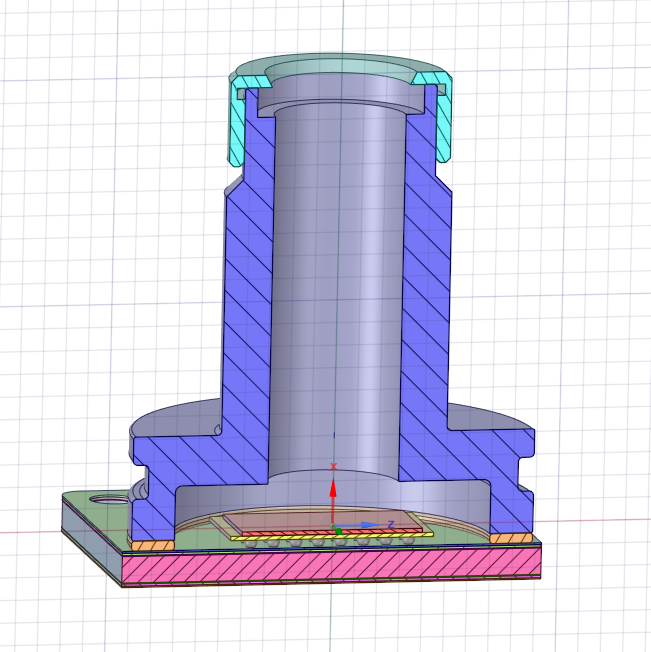nI am performing a static-structural analysis with linear material properties.nThe mesh used is the default mesh that is done by the program.Lets say I start with a default element size of 1.5 mm. I solve the model and I get a value of equivalent stress.nI set up the mesh element size as a paramater and then set the values of this parameter as 1 mm, 0.666 mm, 0.444 mm, 0.296 mm and 0,197 mm.nThe maximum value of the equivalent stress is an output parameter.nWhat I find is that the equivalent stress fluctuates with the mesh refinement i.e it sometimes increases and sometimes decreases.nnCould you please tell me what am I doing wrong in this and what causes this fluctuation?nWhat little knowledge I have of the convergence study tells me that the stress should increase initially and then become constant (or change very little)n
•peteroznewman
Subscriber
Mesh refinement is most efficiently done locally around the region of highest stress. First put a coordinate system near the maximum stress. Then insert a Mesh Sizing control on the solid body using Sphere of Influence which will use the coordinate system you created.nPlease reply with some images of the stress showing the whole body and zoomed in on the region of highest stress and show the mesh.n
•Emadigan
Subscriber
Hi Peter,nThat's a great method to accurately tell the max stress tahnk you, I was just wondering two things -n1) Is the max stress (607) obtained from where the line of best fit intersects the Y axis?n2) Are you able to obtain the optimal element size from this? nThanks!n
•peteroznewman
Subscriber
Arrayn1) Yes, the Exact answer for the stress with a zero element size is where the line of best fit intersects the Y axis.n2) The optimal element size is the largest size that is still very close to the best fit line. Don't use larger sizes because then you can't extrapolate to the exact answer.nn